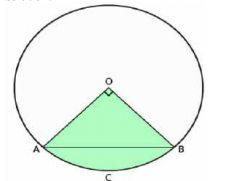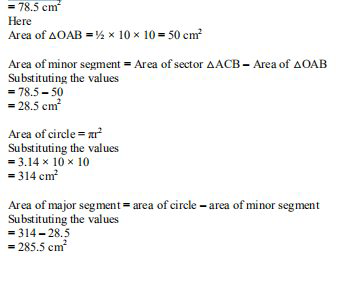# ML Aggarwal Solutions Class 9 Mathematics Solutions for Mensuration Exercise 16.3 in Chapter 16 - Mensuration

Question 22 Mensuration Exercise 16.3

In the figure (i) given below, a chord AB of a circle of radius 10 cm subtends a right angle at the centre

O. Find the area of the sector OACB and of the major segment. Take π = 3.14.It is given that

The radius of the circle = 10 cm

The angle at the center subtended by a chord AB = 90^{\circ}

We know that

Area of sector OACB = πr

2

× 90/360

Substituting the values

= 3.14 × 10 × 10 × 90/360

So we get

= 314 × ¼Video transcript
"hello and welcome my dear students i'm richard purana the maths you don't know lido and i'm here with a new question it says in the figure 1 given below a chord a b of a circle of radius 10 centimeter subtends a right angle at the center o find the area of the sector oacb and of the major segment take pi as 3.14 so be careful while solving the question so without wasting much time let's have a look at the solution so it is given that the radius of the circle is 10 so i'm writing radius for that the radius the angle of this intercept ended by the chord a b it's 90 degree we know that area sector oacb is equals to pi r square into 90 upon 360. which is nothing but equals to 3.14 into one by four so solving this we get c so the radius given as 10 so be careful that you also put the values of the radius which is 1 by 4 so now solving this you get 1 by 4 which is nothing but 78.5 centimeter square so hence the area of oacb is the 78.5 centimeter square right now let's find out the area of triangle oab half into base into height which is half into 10 into 10 being the radius which is 50 centimeter square now the area of the minus segment is equals to area sector oacb minus area of triangle oab which is nothing but equals to 78.5 minus 50 which is nothing but equals to 28.5 centimeter square also the area of circle is pi r square this we all know and pi is 3.14 into radius being 10 so this is equal to 314 centimeter square now hence we need to find out the age of major segment so let's look at that so area of major segment is equals to area of circle minus area of minus segment which is nothing but equals to 314 minus 28.5 which is nothing but equals to 285.5 centimeter square so i hope my students got this question well do not forget to hit the subscribe button you can also share your doubts in the comment section see you next time bye"
Related Questions

Lido

Courses

Teachers

Book a Demo with us

Syllabus

Maths
CBSE
Maths
ICSE
Science
CBSE

Science
ICSE
English
CBSE
English
ICSE
Coding

Terms & Policies

Selina Question Bank

Maths
Physics
Biology

Allied Question Bank

Chemistry
Connect with us on social media!